Contents >> Applied Mathematics >> Numerical Methods >> Interpolation of Functions >> Introduction

 Interpolation of functions - Introduction Introduction Interpolation is an operation of approach of function given in separate points inside of some given interval. The elementary problem of interpolation consists in the following. On a segment [ a , b ] n +1  points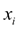( i = 0, 1, 2, …, n ) are given, named nodes of interpolation , and values of some function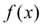in these points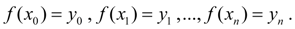It is required to construct the interpolating function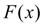accepting in nodes of interpolation the same values, as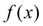, i.e.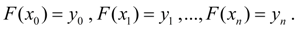Vectorially it means (Fig. 1), that it is required to find some curve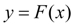of the certain type which are passing through the given set of points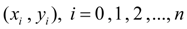.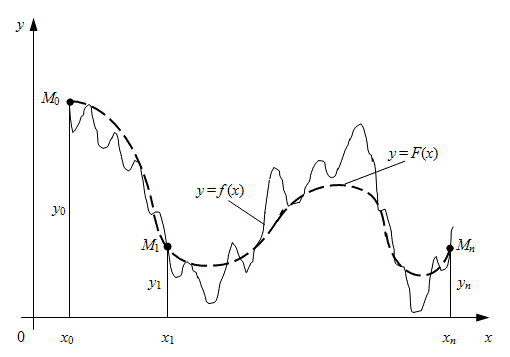Fig. 1. Geometrical representation of function interpolation In such statement the problem of interpolation, generally speaking, can have either uncountable set of solutions or not have at all solutions. However the problem it becomes unequivocal solved if instead of any function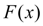to search a polynomial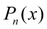of degree not above n , satisfying to conditions: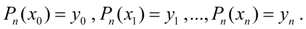(1) Received interpolation formula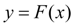is used for approached calculation of values of the given function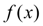for those х which are distinct from nodes of interpolation. Such operation refers to as interpolation of function.

 < Previous Contents Next >